• 很好的提取指定图像中指定颜色的像素点坐标的c++代码
• 获取图片每个像素点的rgb值 源码 自己再封装成类
• ## 图片像素点统计

千次阅读 2019-05-26 14:02:17
这里二分化就是把所有像素点变成纯黑或纯白，因为这张图片在黑白相间处的像素点存在杂色的像素点(毕竟不是矢量图)。 #coding:utf-8 from PIL import Image # 打开图片,convert图像类型有L,RGBA img = ...
最近这几天闲来无事，想起来18年12月末帮别人做了一个编程题。题目就是如何统计一张图片中的气泡数目，以及每个气泡的面积。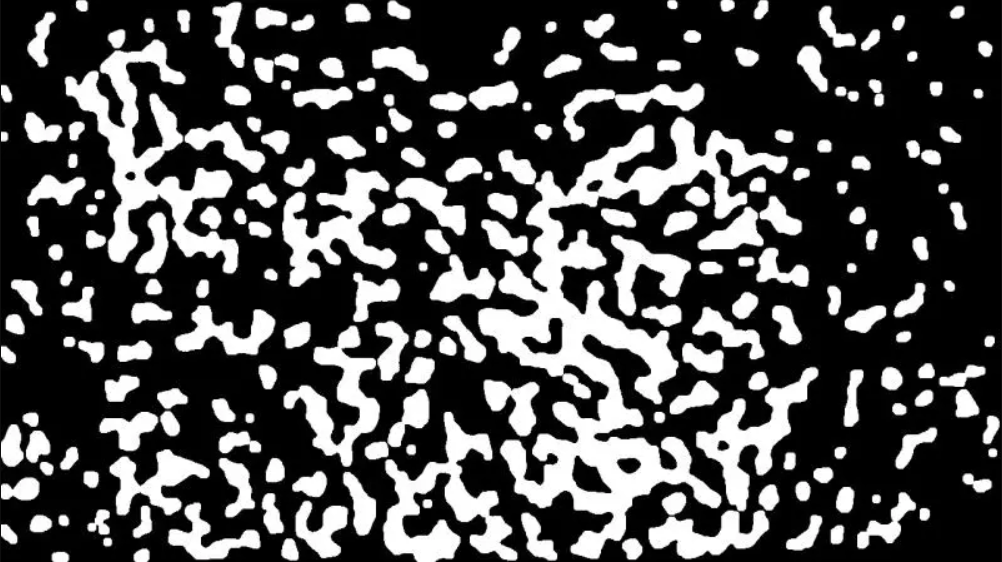上面这张图就是案例，里面白色的都是不规则形态的气泡。当拿到这个题目时看一眼就大致有些思路。因为怎么说也是学了数据结构的人嘛！当时就是想借用数据结构中的“广度优先遍历”算法的思路去做。但是不全是，就是稍微改变一下。不过代码写完加调试估计花了我3个多小时，间接说明我编程能力赶不上我的思维能力。说白了我就菜......
为了给别人理解，我当时就没有采用像素点(三维数据)去直接做，而是多做一步无用功。就是先把像素点降维成为一维数据并且进行简单的二分化。这里二分化就是把所有像素点变成纯黑或纯白，因为这张图片在黑白相间处的像素点存在杂色的像素点(毕竟不是矢量图)。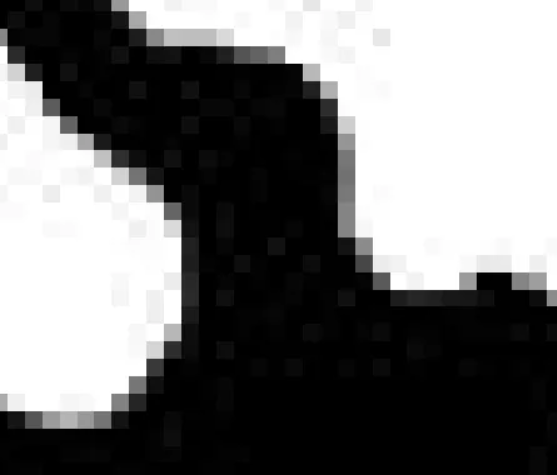#coding:utf-8

from PIL import Image

# 打开图片,convert图像类型有L,RGBA
img = Image.open('1.jpg').convert('L')
img = img.transpose(Image.ROTATE_90)
#img.show();print(img.size,img.size)

# 将黑白图转化为0-1表示
def blackWrite(img):
pixelShift = []
for x in range(img.size):
rowList = []
for y in range(img.size):
# 存在单一像素点不是纯黑纯白,取50为黑白分界线
if img.getpixel((x,y)) < 50:
rowList.append(0)
else:
rowList.append(1)
pixelShift.append(rowList)
return pixelShift
如果把一张图片看成一个二维数组，上面的代码就是把像素点变成0，1存储的二维数组，二维数组的行数和列数就是图片原始的分辨率大小。
然后你就可以把图片类型文件问题转变成我们基本都会的二维数组的字符问题了，剩下来就是如何对二维数组操作了。之前上面提到的“广度优先遍历”，学过数据结构的应该都知道这个图遍历算法。从一个点开始出发，查看它的四周有没有连接点(邻居)，有的话就把它邻居都打入队列中去，然后没有邻居了之后就从队列的队首取出它之前的邻居点，然后查看这个点的邻居(就是邻居的邻居)。之后步骤和上面一样，但是需要对访问过的点做好标记，避免多次重复访问同一个点陷入死循环。
from queue import Queue

# 统计各气泡数字1
def statisticalNum(resultPixel):
bubble = []     # 用于存放各气泡面积
for x in range(len(resultPixel)):
for y in range(len(resultPixel)):
if resultPixel[x][y] == 1:
resultPixel[x][y] = 0
Coordinate = Queue()    # 存放该点四周为1的坐标点
onlyCoordinate = []     # 防止重复的坐标点入队,并统计个数
Coordinate.put([x,y])
while not Coordinate.empty():
row = clo = 0
[row,clo] = Coordinate.get()
onlyCoordinate.append([row,clo])
# 查看左边坐标点
if clo > 0:
if (resultPixel[row][clo-1] == 1) and ([row,clo-1] not in onlyCoordinate):
Coordinate.put([row,clo-1])
resultPixel[row][clo-1] = 0
# 查看右边坐标点
if clo < (img.size-1):
if (resultPixel[row][clo+1] == 1) and ([row,clo+1] not in onlyCoordinate):
Coordinate.put([row,clo+1])
resultPixel[row][clo+1] = 0
# 查看下边坐标点
if row < (img.size-1):
if (resultPixel[row+1][clo] == 1) and ([row+1,clo] not in onlyCoordinate):
Coordinate.put([row+1,clo])
resultPixel[row+1][clo] = 0
bubble.append(len(onlyCoordinate))
return bubble

我的思路：

从第0行开始遍历，遇到第一个1(白色像素点)时，开始对其左，右，下三个方向数组点值做判断，周围邻居值是1就说明它们都在同一个气泡中，然后把邻居们压入队列中。  当这个点访问完成后，需要这个点的值1置为0表示访问完毕，避免它右邻居点在访问时把左边的它压入队列再次访问，会使得这个气泡的面积变大，甚至是死循环。(不要问我为什么这么清楚，呜呜呜呜呜)  把置0点的左边存在一个list列表中，类似c/java中的数组类型。  当第一层循环结束时得到了一个气泡，只需要用len方法就知道这个气泡点的个数，即面积。  然后第二次循环时，二维数组中的1减少一部分；即使代码是3个循环嵌套，也不要说我的代码时间复杂度是O(n^3)。

关于时间复杂度，我从来都喜欢用书本上的公式来衡量一个算法好坏。我就喜欢用实际中它运行的真实次数和时间来衡量一个算法的好坏。这么说吧，书上喜欢把O(n^2)+O(n)当成O(n^2)来衡量，忽略O(n)。如果在实际中n比较大的时候，O(n)消耗的资源成本也要考虑到的。想成为一个好的程序员就要比别人多注意一些细节，在大数据时代更是如此。
项目源码和图片的链接在此：https://gitee.com/mjTree/MyPython/tree/master/图片气泡统计银长臂猿
银长臂猿?：栖息在热带及亚热带的雨林和密林，树栖性、日行性。生活在高大的树林中，采用“臂行法”行动，像荡秋千一样从一棵树到另一棵树，一次可跨越3米左右，加上树枝的反弹力可以达8-9米，且速度惊人。但是它们在地面上却显得十分笨拙。食性：杂食性，以树叶、水果、果实、花苞为食。
由于爪哇岛人口的增长，而雨林正在迅速消失中。只有百分之四的银长臂猿的栖息地仍然存在。除了gununghalimun国家公园可能生活着1000只以上的银长臂猿，其他地区只有分散的小种群，很难维持其生存繁衍，目前野生的银长臂猿数量不满2000。

展开全文深度遍历 图像识别
• 一、遍历访问图片每个像素点，并修改相应的RGB import cv2 as cv def access_pixels(image): print(image.shape) height = image.shape width = image.shape channels = image.shape print(&...
一、遍历图片三个通道像素点，并修改相应的RGB
def access_pixels(image):
print(image.shape)
height = image.shape
width = image.shape
channels = image.shape
print("width: %s  height: %s  channels: %s"%(width, height, channels))
for row in range(height):
for col in range(width):
for c in range(channels):
pv = image[row , col, c]              #获取每个像素点的每个通道的数值
image[row, col, c]=255 - pv           #灰度值是0-255   这里是修改每个像素点每个通道灰度值
cv2.imshow("Reverse_phase_image",image)

if __name__ =="__main__":
cv2.imshow('original_image', src)                  #显示图像

t1 = cv2.getTickCount()                            #GetTickcount函数返回从操作系统启动到当前所经过的毫秒数
access_pixels(src)
t2 = cv2.getTickCount()
time = (t2-t1)/cv2.getTickFrequency()              #getTickFrequency函数返回CPU的频率,就是每秒的计时周期数
print("time : %s ms"%(time*1000) )                 #输出运行时间
cv2.waitKey(0)
cv2.destroyAllWindows()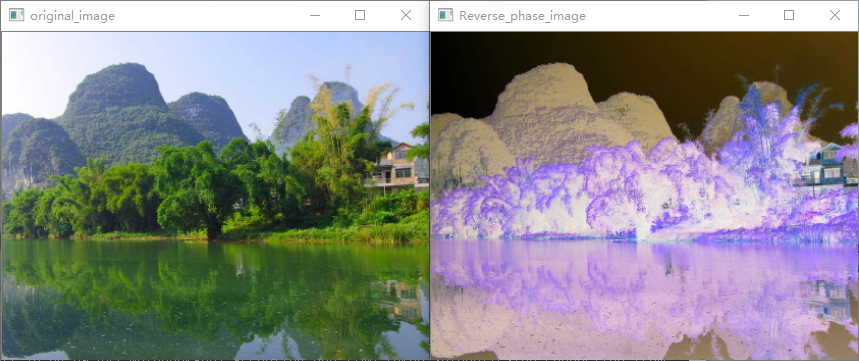测试如何高效遍历像素
我本来想如何加速opencv-python的遍历像素效率，唯一能够稍微加快的方式是：先遍历小的数据范围，然后遍历大的数据范围。这样才能使遍历的速度有所加快。也就是说以下两种方式效果一样。
通过下述代码发现，最好不要通过遍历像素来操作图像，我们可以用numpy的矩阵运算。 若是C++代码，可以使用C语言的指针来遍历，通过指针操作像素的地址来操作对应数字会大大加速运算。
import cv2
import time
import numpy as np

# 遍历依次为通道，高，宽。
def inverse(image):
height = image.shape
width = image.shape
channels = image.shape
pixel_data = np.array(image, dtype = np.uint8)
for c in range(channels):
for row in range(height):
for col in range(width):
level = pixel_data[row, col, c]
pixel_data[row, col, c] = 255 - level
return pixel_data

def inverse2(image):
height,width = image.shape[:2]
channels = image.shape
for c in range(channels):
for row in range(height):
for col in range(width):
level = image[row, col, c]
image[row, col, c] = 255 - level
return image

if __name__ == '__main__':
imgpath = r'C:\Users\xxxx\Desktop\201920100013253001_30302_01.jpg'
print(image.shape)
print(image.size)
print(image.dtype)

start1 = time.time()
image1 = inverse(image)
end1 = time.time()

start2 = time.time()
image2 = inverse2(image)
end2 = time.time()

print('cost_time1:',end1 - start1)
print('cost_time2:', end2 - start2)
cv2.imshow("inverse1 image", image1)
cv2.imshow("inverse2 image", image2)
cv2.waitKey(0)
cv2.destroyAllWindows()
'''
(1732, 2309, 3)
11997564
uint8
cost_time1: 17.330647706985474
cost_time2: 17.001970291137695

Process finished with exit code 0
'''

二、非赋值修改像素
def get_bin_table(threshold=140):  #二值化图片（非函数，直接操作像素，简便快速）
table = []
for i in range(256):
if i < threshold:
table.append(0)
else:
table.append(1)
return table

去除二值化后孤立点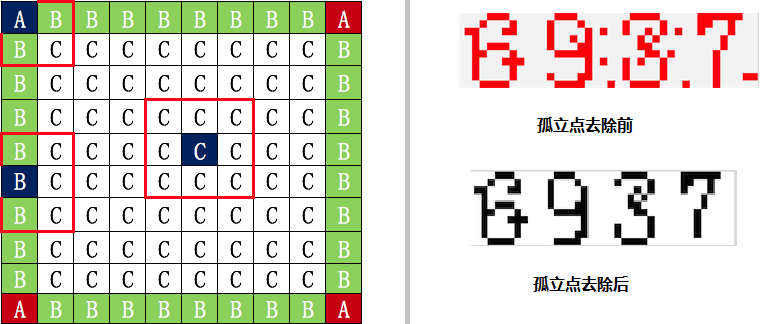def sum_9_region(img, x, y):
"""
9邻域框,以当前点为中心的田字框,黑点个数
:param x:
:param y:
:return:
"""
# todo 判断图片的长宽度下限
cur_pixel = img.getpixel((x, y))  # 当前像素点的值
width = img.width
height = img.height

if cur_pixel == 1:  # 如果当前点为白色区域,则不统计邻域值
return 0

if y == 0:      # 第一行
if x == 0:  # 左上顶点,4邻域
# 中心点旁边3个点
sum = cur_pixel \
+ img.getpixel((x, y + 1)) \
+ img.getpixel((x + 1, y)) \
+ img.getpixel((x + 1, y + 1))
return 4 - sum
elif x == width - 1:  # 右上顶点
sum = cur_pixel \
+ img.getpixel((x, y + 1)) \
+ img.getpixel((x - 1, y)) \
+ img.getpixel((x - 1, y + 1))

return 4 - sum
else:  # 最上非顶点,6邻域
sum = img.getpixel((x - 1, y)) \
+ img.getpixel((x - 1, y + 1)) \
+ cur_pixel \
+ img.getpixel((x, y + 1)) \
+ img.getpixel((x + 1, y)) \
+ img.getpixel((x + 1, y + 1))
return 6 - sum
elif y == height - 1:  # 最下面一行
if x == 0:  # 左下顶点
# 中心点旁边3个点
sum = cur_pixel \
+ img.getpixel((x + 1, y)) \
+ img.getpixel((x + 1, y - 1)) \
+ img.getpixel((x, y - 1))
return 4 - sum
elif x == width - 1:  # 右下顶点
sum = cur_pixel \
+ img.getpixel((x, y - 1)) \
+ img.getpixel((x - 1, y)) \
+ img.getpixel((x - 1, y - 1))

return 4 - sum
else:  # 最下非顶点,6邻域
sum = cur_pixel \
+ img.getpixel((x - 1, y)) \
+ img.getpixel((x + 1, y)) \
+ img.getpixel((x, y - 1)) \
+ img.getpixel((x - 1, y - 1)) \
+ img.getpixel((x + 1, y - 1))
return 6 - sum
else:  # y不在边界
if x == 0:  # 左边非顶点
sum = img.getpixel((x, y - 1)) \
+ cur_pixel \
+ img.getpixel((x, y + 1)) \
+ img.getpixel((x + 1, y - 1)) \
+ img.getpixel((x + 1, y)) \
+ img.getpixel((x + 1, y + 1))
return 6 - sum
elif x == width - 1:  # 右边非顶点
# print('%s,%s' % (x, y))
sum = img.getpixel((x, y - 1)) \
+ cur_pixel \
+ img.getpixel((x, y + 1)) \
+ img.getpixel((x - 1, y - 1)) \
+ img.getpixel((x - 1, y)) \
+ img.getpixel((x - 1, y + 1))

return 6 - sum
else:  # 具备9领域条件的
sum = img.getpixel((x - 1, y - 1)) \
+ img.getpixel((x - 1, y)) \
+ img.getpixel((x - 1, y + 1)) \
+ img.getpixel((x, y - 1)) \
+ cur_pixel \
+ img.getpixel((x, y + 1)) \
+ img.getpixel((x + 1, y - 1)) \
+ img.getpixel((x + 1, y)) \
+ img.getpixel((x + 1, y + 1))
return 9 - sum

展开全文• import cv2 # 读取图像 img = cv2.imread("test.jpg") # 查看图像 cv2.imshow("title", img) cv2.waitKey(0) # 查看维度 print(img.shape) ...# 查看 (10,10) 位置的RGB # 注意：此处返回顺序为 [B, G, R] ...
import cv2

# 读取图像

# 查看图像
cv2.imshow("title", img)
cv2.waitKey(0)

# 查看维度
print(img.shape)

# 查看 (10,10) 位置的RGB
# 注意：此处返回顺序为 [B, G, R]
print(img[10,10])

# 更改 部分为白色
for i in range(100):
for j in range(100):
img[i, j] = [200,255,255]

# 保存
cv2.imwrite("test2.png", img)   # 用 imwrite 函数将数据写到本地

展开全文openCV
• ## 图片像素点获取

千次阅读 2020-07-18 14:36:10
在很多时候进行图片处理深度学习都需要知道坐标，再此以获取照片的地址做一个例子，讲述一种通过鼠标获取像素点的方法 必须清楚：这个方法只能在python idle上实现，不能用jupyter notebook打开的（亲测过）。 ...
在很多时候进行图片处理深度学习都需要知道坐标，再此以获取照片的地址做一个例子，讲述一种通过鼠标获取像素点的方法 必须清楚：这个方法只能在python idle上实现，不能用jupyter notebook打开的（亲测过）。
import os , re
import numpy as np
img = Image.open(save_path+'/0.jpg')
print(img.size)
image_arr = np.array(img)
imshow(image_arr)
x =ginput(4)
print ('you clicked:',x)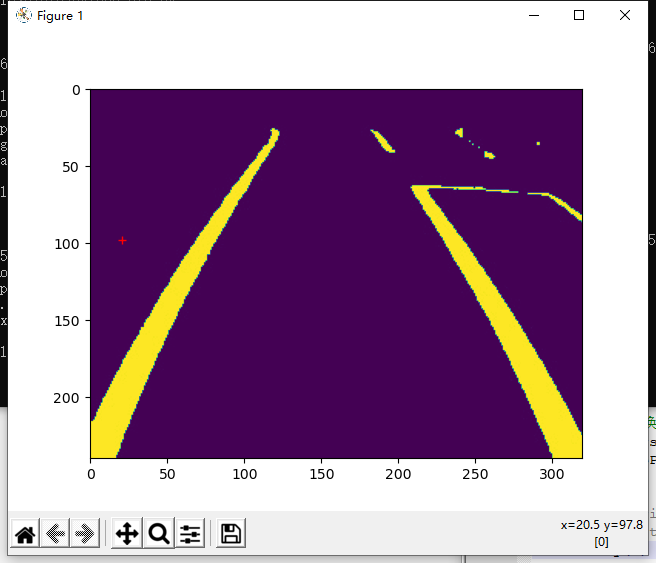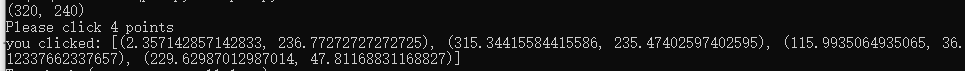参考链接
展开全文python opencv 深度学习
• Qt载入图片界面，并通过鼠标移动时间，获得图片上每个像素点坐标。
• python中除了使用PIL库处理图像之外还可以使用CV2 import cv2 import numpy as np fileName = '2.png' print(fileName) img =...dst) 结果图： 使用PIL库遍历图片以及改变颜色详见上篇博客： PIL遍历像素以及改变像素opencv python 计算机视觉
• 仅仅为了简单地熟悉像素处理方法 做的是14年的建模A题嫦娥登陆。 其中一个问题是这样的 如何选择下面这个图（月球表面高度2400米处的照片）的嫦娥三号着陆？ 其实官方给的数据里有图像中每个...
• 1 基于图像单像素点的处理 看过数字图像处理一书的都知道，图像处理中基于像素点的处理分为两种 灰度变换：本质就是基于单像素点的变化处理。 空间滤波：本质就是基于邻域像素点的变化处理。 今天要讲的是在OpenCv下...灰度变换 OpenCv
• 在有些情况下，我们需要对屏幕上的像素点进行大量的绘制操作。比如我之前模拟写的一个渲染管线开源练习，涉及到了大量的像素点操作。而Qt本身的QPen和QPainter::drawPoint的API如果操作大量的像素点，会非常耗时，...Qt
• from PIL import Image # 打开要处理的图像 img_src = Image.open('sun.png') # 转换图片的模式为...# 获得文字图片的每个像素点 src_strlist = img_src.load() # 100,100 是像素点的坐标 data = src_strlist[...
• 图像中，已知了某连通域的一个像素点，如何根据该像素点确定像素点所在的连通域(比如图像中有多个连通域，而现在只知一个连通域的像素点，如何根据该点反推像素点所在的连通域，并标记出来)图像处理 算法
• python中使用PIL库对图片像素点遍历以及像素值改变将图片中特定像素值的部分改为另一种像素值读取图片的像素值改变颜色实验图片展示完整代码 将图片中特定像素值的部分改为另一种像素值 实验目的：将一张图片中白色...python 计算机视觉 opencv
• 1、matlab获取彩色图像像素值的方法 img=imread('test.jpg'); img1=img(:,:,1); img2=img(:,:,2); img3=img(:,:,3); 三通道排序分别对应彩色图像的rgb三通道 2、opencv中获取图像像素的方法 方法一： IplImage *img ...
• 图像的基本单元是一个像素，就像一个巨幅电子广告屏上远处看是衣服图像，走近你会看到一个一个的方格，这个方格的颜色是一种，从远处看，觉察不到这个方格的存在。 一个像素需要3块表示，分别代表R，G，B，如果8为...RGB
• OpenCV像素点邻域遍历效率比较，以及访问像素点的几种方法opencv 遍历 运行效率
• 一张图片放大以后就会呈现一个个的点阵，每一个点就是一个像素点，通过我们对RGB的颜色配比就可以显示出不同的颜色效果了，所以说最终我们想对一个图像进行处理的话就是对一个个像素点进行处理。图像处理 android
• PYTHON CV2 图片中鼠标点击获取像素点坐标
• 它提供了许多常用的图像处理算法相关的函数，极大地方便了图像处理方法的开发，而图像处理最本质的就是对图像中像素点的像素值的运算。所以我们需要了解一下OpenCV如何访问图像中的像素点。首先说明一下，这里默认...OpenCV
• Java OpenCV-4.0.0 图像处理8 图像像素点的获取与操作 java OpenCV-4.0.0 获取图像像素点并对像素点进行操作 /** * OpenCV-4.0.0 获取图像像素点 */ public static void getPixelImage() { Mat src = Imgcodecs....Java OpenCV 图像处理
• 一. 所需结构体CvScalar结构体介绍typedef struct ... 所需函数cvGet2D函数功能：获取像素点上的RGB颜色分量，如果是灰度图直接返回灰度图颜色分量函数原型：CvScalar cvGet2D( const CvArr* arr, int idx0, int idx...opencv CvScalar cvGet2D
• 虽然使用OpenCV已经有一段时间，但是一直没有涉及单个像素点的操作。今天在提取像素点绘制水平以及数值轴直方图时，总感觉直方图提取反了，直到x,y坐标交换才得出正确结果。 找到这篇文章，坐标系以及像素点的提取...opencv
• #对图片的像素进行读写操作 ...4.图片的宽和高 “640*480”表示图片在水平方向上有640个像素点，在竖直方向上有480个像素点 5.未压缩的图片的计算方法： 1.14M（图片的大小）=720*547（图片像素点的个数）*3（颜色通...
• 多日的单片机，让我心烦，今天写一个获取像素点信息的程序，查百度。呵呵 并没有发现什么有用的信息，还是走老本行吧。/******************************************************************** ** 文 件 名：像素点...c rgb
• 从画布上获取指定区域的像素点，作为一个新的ImageData对象（可以将照片放到画布上，然后获取照片的指定区域） （x，y）为左上角起点坐标，width和height为指定区域的大小，可以为一个像素 3、putImageData(object,x...
• matlab标注图片中的坐标为（850，593）像素点的位置，用圆圈标注且圆圈大小可调。 参考，感谢优秀博主： 传送门1 传送门2 代码 clear;clc;close all; I=imread('E:\code\findlocation.png'); [a,b]=size(I) imshow...
• 实现原理很简单，扫描图片像素点，识别出RGB数值后，转成单位格颜色填充到excel里。 由于涉及到大量计算，图片最好不要超640480像素。* 废话不多说，直接上代码 from PIL import Image import openpyxl from ...python 图像识别
• 生成黑白图像 data=[172 179 184 184 178 178 177 173 171 174 177 178 176 169 165 161 163 161 180 205 201 183 171 177 178 180 194 101 55 65 60 47 55 65 63 59 58 63 57 52 90 105 117 122 130 143 153 157 ...图像处理
• 【webkit的源码】https://github.com/WebKit/webkit/blob/master/Source/WebCore/html/HTMLCanvasElement.cpp#L365 ...这意味着 一个逻辑像素需要9个物理像素去补充。那就意味着 dpr=3的一个1px（逻辑）像素需要 9......